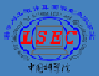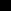###Academic Staff of LSEC:

#### Professor:Zhong-Zhi Bai   Numerical linear algebra, Parallel computationsLi-qun Cao   Multiscale computationZhiming Chen   Finite element method, Maxwell equations,
Flow transport in porous mediaJun-zhi Cui   Multiscale computaion for composite materials, Scientific computingTao Cui   Parallel computing, electromagnetic computingXiao-ying Dai   Finite element method, eigenvalue problemYu-hong Dai   Nonlinear optimizationJia-lin Hong   Structure-preserving algorithms for dynamical systemsQi-ya Hu   Integral equation, domain decompositionXing-biao Hu   Soliton theory and integrable systemsQun Lin   Finite element method, superconvergenceXin Liu   Computational Methods for OptimizationBen-zhuo Lu   Biological computation and simulationShi-peng Mao   Finite element methods, Agaptive methods,
Computational fluid and magneto-fluid dynamicsPing-bing Ming   Finite element methods, Nonconforming methods, Multiscale
modelling and computationYa-juan Sun   Geometric Numerical Integration for ODEs and PDEsYi-fa Tang   Symplectic Algorithms for Hamiltonian Systems, Numerical Analysis
of Fractional Differential Equations with Applications
Machine Learning and Dynamical SystemsHe-hu Xie   Numerical methods for partial differential equations,
eigenvalue problems and integral-differential equationsXue-jun Xu   Finite element method, domain decompositionZhi-qiang Xu   Approximation theory, Data Sciences and Computational Harmonic AnalysisHai-jun Yu   Complex fluids, numerical methods for high dimensional problems,
spectral methodLi Yuan   Computational Fluid DynamicsYa-xiang Yuan   Nonlinear optimizationLin-bo Zhang   Parallel computingWen-sheng Zhang   Inverse problemWei-ying Zheng   Finite element method, Electromagnetic and optical computationAihui Zhou   Finite element method, eigenvalue problem, chaotic dynamicsTao Zhou   Stochastic Computations, Uncertainty Quantification

#### Associate Professor:Xiangke Chang   Numerical algorithms, Approximation problems and Inverse
problems related to Integrable systemsChong Chen   Biomedical Imaging, Image Processing,Visualization
in Scientific ComputingChuchu Chen   Structure-preserving algorithms for stochastic

(partial) differential equationsBin Gao   Optimization on manifoldsWei Gong   Numerical methods for optimal control problems,
finite element methodJi-zu Huang

Multiscale model and parallel algorithmWei Leng

Numerical methods for incompressible Navier-Stokes

equations,Seismic ImagingWenbo Li   Numerical methods for PDEs, Fully nonlineare PDEs, nonlocal PDEsJiulong Liu   Computational inverse problems, Medical image reconstruction, Machine learningYa-feng Liu   Optimization algorithm and its applications to wireless communicationsYong Liu   Computational Fluid Dynamics, Discontinuous Galerkin methodsJunjie Ma   Signal processing, probabilistic graphical model, optimization algorithms and applicationsBin Shi   Optimization, machine learning, and differential equationXu Wang   Stochastic inverse problems for PDEs, Numerical methods for stochastic PDEsJie Xu   Modeling and simulation of liquid crystals,numerical methods for gradient flowsXian-min Xu   Computational materials, multi-scale methodsTao Yin   Boundary integral equation, Direct and inverse scattering problemChen-song Zhang   Adaptive finite element methods, multigrid methodsShuo Zhang   Finite element methods, fast solvers for linear systems

#### Assistant Professor:###Supporting Staff of LSEC:Ru-juan Ding   SecretaryJin Chen   SecretaryYing Liu   SecretaryYing Qian   Computer System AdministratorUpdated March 2023